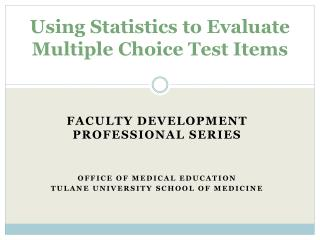Download PresentationUsing Statistics to Evaluate Multiple Choice Test Items

# Using Statistics to Evaluate Multiple Choice Test Items

Download Presentation## Using Statistics to Evaluate Multiple Choice Test Items

- - - - - - - - - - - - - - - - - - - - - - - - - - - E N D - - - - - - - - - - - - - - - - - - - - - - - - - - -
##### Presentation Transcript

1. Using Statistics to Evaluate Multiple Choice Test Items Faculty Development Professional Series Office of Medical Education Tulane University School of Medicine

2. Objectives • Define test reliability • Interpret KR-20 statistic • Evaluate item difficulty (p value) • Define and interpret the point biserial correlation • Evaluate distractor quality • Differentiate among poor, fair, and good test items

3. What is Reliability? • Test reliability is a measure of the accuracy, consistency, or precision of the test scores. • Statistics: • Coefficient (Cronbach) Alpha – generally used for surveys or tests that have more than one correct answer • Kuder-Richardson Formula (KR-20) – Measures inter-item consistency or how well your exam measures a single construct. Used for knowledge tests where items are scored correct/incorrect (dichotomous) • KR-21 – Similar to KR-20 but underestimates the reliability of an exam if questions are of varying difficulty

4. Interpreting KR-20 • KR-20 statistic is influenced by: • Number of test items on the exam • Student performance on each item • Variance for the set of student test scores • Range: 0.00 – 1.00 • Values near 0.00 – weak relationship among items on the test • Values near 1.o0 – strong relationship among items on the test • Medical School exams should have a KR-20 of .70 or higher

5. Improving Reliability Reliability can be improved by: Writing clear and simple directions Ensuring test items are clearly written and follow NBME guidelines for construction Assuring that test items match course objectives and content Adding test items; longer tests produce more reliable scores

6. Item Difficulty • Item Difficulty (p value) – measure of the proportion of students who answered a test item correctly • Range – 0.00 – 1.00 • Ex. p value of .56 means that 56% of students answered the question correctly. • p value of 1.00 means that 100% of students answered the question correctly. • For medical school tests where there is an emphasis on mastery, MOST items should have a p-value of .70 or higher.

7. What is a point biserial correlation? The point biserial correlation: • Measures test item discrimination • Ranges from -1.00 to 1.00 • A positive point biserial indicates that those scoring high on the total exam answered a test item correctly more frequently than low-scoring students. • A negative point biserial indicates low scoring students on the total test did better on a test item than high-scoring students. • As a general rule, a point biserial of ≥.20 is desirable.

8. Distractor Analysis • Addresses the performance of incorrect response options. • Incorrect options should be plausible but incorrect. • If no one chooses a particular option, the option is not contributing to the performance of the test item • The presence of one or more implausible distractors can make the item artificially easier than it should be.

9. Point biserial analysis • Items that are very easy or very difficult will have low ability to discriminate. • Such items are often needed to adequately sample course content and objectives. • A negative point biserial suggests one of the following: • The item was keyed incorrectly. • The test item was poorly constructed or was misleading. • The content of the item was inadequately taught.

10. Test Item Analysis Example 1 Interpretation: • Correct answer is A • p value = 83.51 • question answered correctly by 83.51% of class • point biserial of .40 • ≥.20 – high scoring students were more likely to choose the correct answer • all distractors chosen • GOOD QUESTION

11. Test Item Analysis Example 2 • Interpretation: • Correct answer is C • p value = 25.57 • question answered correctly by only 25.57% of class • point biserial = -0.14 • <.20 – low scoring students were more likely to choose the correct answer • POOR QUESTION

12. Test Item Analysis Example 3 • Interpretation: • Correct answer is D • p value = 97.73 • question answered correctly by 97.73% of class • Point biserial = 0.08 • <.20 – BUT almost all students answered correctly so item is unable to discriminate. This is okay if item tests a concept all students are expected to know. • FAIR QUESTION

13. Self-Assessment Review the following test item statistics: What can you conclude about this test item? Click your response below. The distractors were implausible and should be replaced. Low scoring students got this item correct more often than high-scoring students. More than 10% of the class answered this question incorrectly. This test item showed high discriminative ability and should be retained.

14. Summary